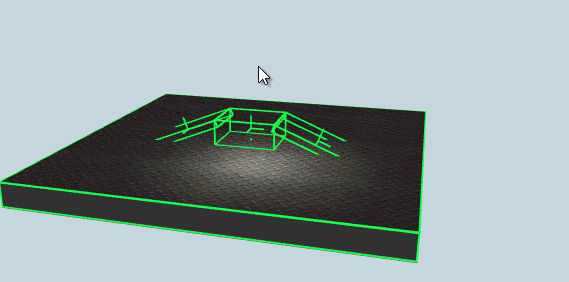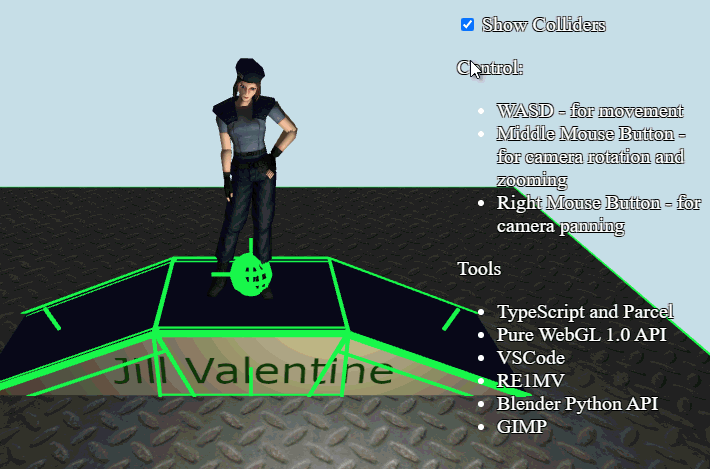# Is it possible to get the Bullet's drawLine function for a custom renderer?

Hi,

I want to draw colliders with my own renderer in OpenGL3 and PyQt6. The drawLine method is in private member DebugDraw _drawer; Can I get the drawLine function to draw the collder’s segments?

It’s not entirely clear what you’re trying to do. However, I think it’s not difficult to visualize primitives by just getting their sizes. As for polygons, you can always get the model grid data.

I already made visualizing of colliders by primitives (I have a problem with size of ground collider, but it does not matter):But Bullet Physics has a very simple method to draw segments of colliders. It has the btIDebugDraw class with the drawLine method:

``````virtual void drawLine (
const btVector3 &from,
const btVector3 &to,
const btVector3 &color)=0
``````

I use a cube for the edge with lightless shader. Coordinates of segment was given by arguments above (`from` and `to`):I just transform this cube in my class:

``````import Ammojs from "ammojs-typed";
import { Ammo, physicsWorld } from "./ammojs-init";
import { mat4, quat, vec3 } from "gl-matrix";
import ColliderEdge from "./collider-edge";

export default class DebugDrawer
{
public projMatrix: mat4;
public viewMatrix: mat4;

private _edgeObject: ColliderEdge;
private _debugDrawer: Ammojs.DebugDrawer;
private _unitX = vec3.fromValues(1, 0, 0);
private _tempVec = vec3.create();
private _projViewMatrix = mat4.create();

public constructor(edgeObject: ColliderEdge)
{
this._edgeObject = edgeObject;
this._debugDrawer = new Ammo.DebugDrawer();

this._debugDrawer.drawLine = (from: any, to: any, color: any): void =>
{
const heap = Ammo.HEAPF32;
const r = heap[(parseInt(color) + 0) / 4];
const g = heap[(parseInt(color) + 4) / 4];
const b = heap[(parseInt(color) + 8) / 4];

const fromX = heap[(parseInt(from) + 0) / 4];
const fromY = heap[(parseInt(from) + 4) / 4];
const fromZ = heap[(parseInt(from) + 8) / 4];

const toX = heap[(parseInt(to) + 0) / 4];
const toY = heap[(parseInt(to) + 4) / 4];
const toZ = heap[(parseInt(to) + 8) / 4];

let centerX: number;
let centerY: number;
let centerZ: number;

if (fromX > toX)
{
centerX = toX + Math.abs(fromX - toX) / 2;
}
else
{
centerX = fromX + Math.abs(toX - fromX) / 2;
}

if (fromY > toY)
{
centerY = toY + Math.abs(fromY - toY) / 2;
}
else
{
centerY = fromY + Math.abs(toY - fromY) / 2;
}

if (fromZ > toZ)
{
centerZ = toZ + Math.abs(fromZ - toZ) / 2;
}
else
{
centerZ = fromZ + Math.abs(toZ - fromZ) / 2;
}

this._tempVec = toX - fromX;
this._tempVec = toY - fromY;
this._tempVec = toZ - fromZ;
const length = vec3.length(this._tempVec);
vec3.normalize(this._tempVec, this._tempVec);

quat.rotationTo(this._edgeObject.rotation, this._unitX, this._tempVec);
this._edgeObject.scale = length;
this._edgeObject.scale = 0.1;
this._edgeObject.scale = 0.1;
this._edgeObject.position = centerX;
this._edgeObject.position = centerY;
this._edgeObject.position = centerZ;

mat4.mul(this._projViewMatrix, this.projMatrix, this.viewMatrix);
this._edgeObject.draw(this._projViewMatrix);
};

this._debugDrawer.setDebugMode = (debugMode: number) => { }

let debugMode = 1; // 0 - 0ff, 1 - on
this._debugDrawer.getDebugMode = (): number =>
{
return debugMode;
}

this._debugDrawer.drawContactPoint = (pointOnB: Ammojs.btVector3, normalOnB: Ammojs.btVector3,
distance: number, lifeTime: number, color: Ammojs.btVector3): void => { }

this._debugDrawer.reportErrorWarning = (warningString: string): void => { };
this._debugDrawer.draw3dText = (location: Ammojs.btVector3, textString: string): void => { };

this._debugDrawer.setDebugMode(0);
physicsWorld.setDebugDrawer(this._debugDrawer);
}
}
``````

Panda3D has a good port of Bullet Physics. It is only one port in the World. PyBullet does not allow to use a custom OpenGL renderer. But Panda3D hides the DebugDraw class. I think Panda3D does not allow to change these settings:

``````    m_pCollisionConfiguration = new btDefaultCollisionConfiguration();
m_pDispatcher = new btCollisionDispatcher(m_pCollisionConfiguration);
m_pOverlappingPairCache = new btDbvtBroadphase();
m_pSolver = new btSequentialImpulseConstraintSolver();
m_pWorld = new btDiscreteDynamicsWorld(m_pDispatcher, m_pOverlappingPairCache,
m_pSolver, m_pCollisionConfiguration);
``````

But if it will it will awesome for Python community. Make Panda3D Bullet Physics more independent on Panda3D Renderer.

This is my demo in pure WebGL and Ammo.js in TypeScript (it is port of Bullet Physics to JavaScript and TypeScript)